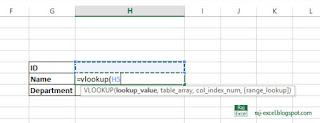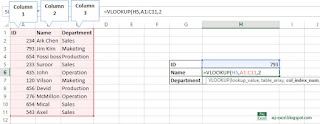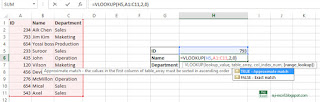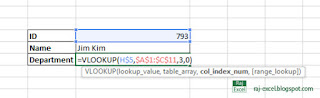d

## Using VLOOKUP() function (step by step tutorial)

How to use VLOOKUP() function in Excelusing VLOOKUP function

Solution of this problem.
You can use the VLOOKUP function to search the first column of a range of cells, and then return a value from any cell on the same row of the range.
Syntax: VLOOKUP (lookup_value, table_array, col_index_num, [range_lookup])
The VLOOKUP function syntax has the following arguments:
lookup_value: Required. The value to search in the first column of the table or range.
table_array: VLOOKUP returns the #N/A error value.
col_index_num: Required. The column number in the table_array argument from which the matching value must be returned range_lookup: Optional. A logical value that specifies whether you want VLOOKUP to find an exact match or an approximate match:Let’s start to using the VLOOKUP function
First we create a list with contacting employee ID, name and departmentType ID number in the column to find the name and department
For example, when we type the ID #. 793 and the name and department of Jim Kim will automatically appearIn H6 cell type the =Vlookup(H5,
(H5) cell [LOOKUP_VALUE] search the data you want to find in the dataSelect the data from A1 to C11
That is the [TABLE_ARRARY] you find the data of ID (H6)Now type 2 [COL_INDEX_NUM] that is the column index number 1 is the column A that contain the ID 2 is the B column that contain the name and 3 is the C column that contain the department.
Here we select the column 2 for find the name of the specific ID.And finally after you have selected the column index just type the 0 or FALSE (it’s for exact match the lookup data).
And press enter key.Type the ID in cell H5 793
You will see the Name “Jim Kim” of ID 793
Copy the formula and paste it to Department and change the column index number 3 and press enter.Now you see the result of ID 793 name and department.